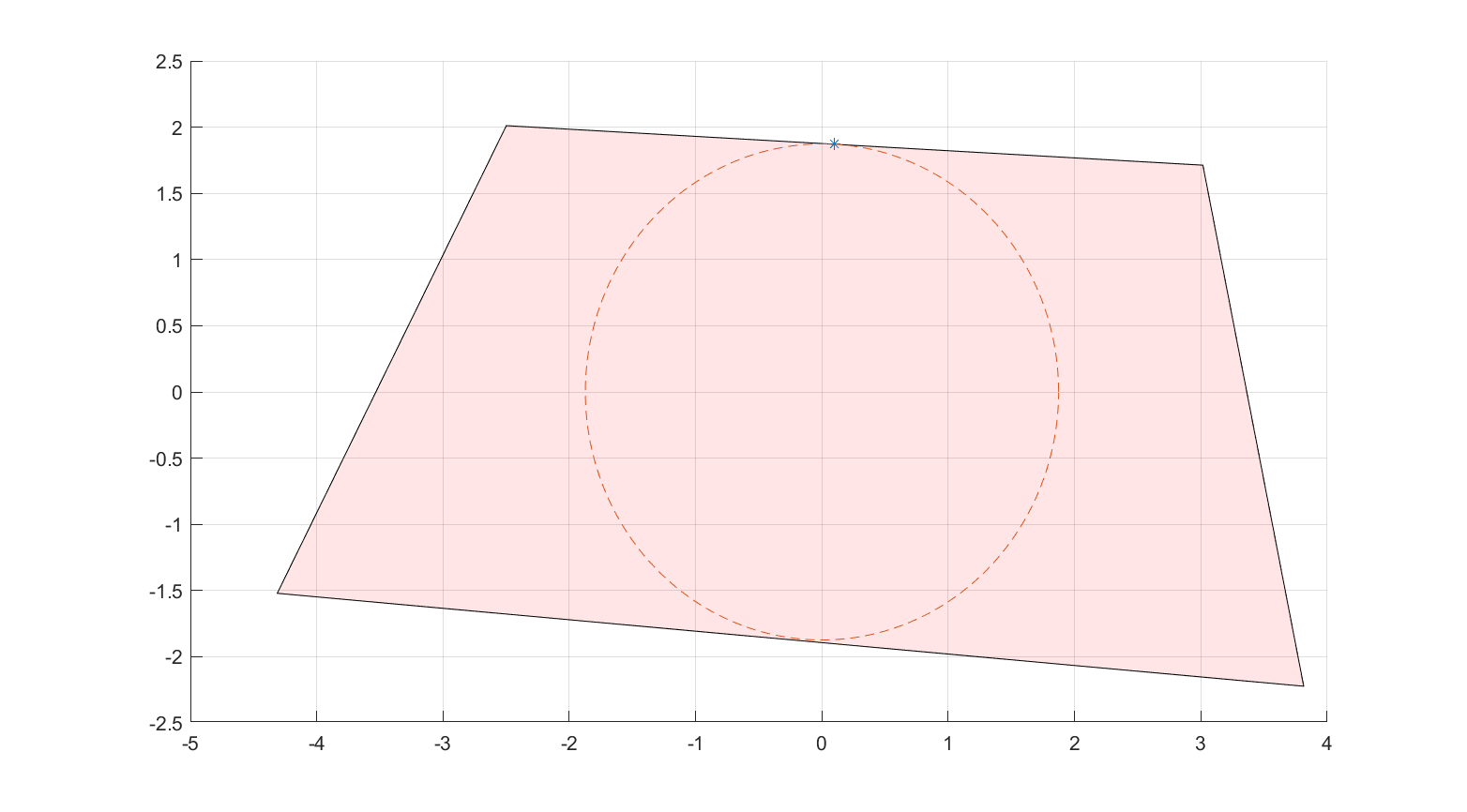# isoutside

Tags:

Updated:

isoutside creates a big-M model to avoid a region specified by linear inequalities.

## Syntax

Model = isoutside(P)


## Example

The following code finds the vertex/point on facet closest to the origin in a polytope by solving a non-convex problem involving an avoidance constraint. Note the required explicit bounds, as the model is constructed using big-M strategies.

x = sdpvar(2,1);
A = randn(10,2);
b = 10*rand(10,1);

Model = isoutside(A*x <= b)

optimize([Model, -100 <= x <= 100],x'*x)
clf